April 28, 2016

IPython Notebook on GPS Timing and CDMA

In :
%matplotlib inline

import matplotlib
import numpy as np
import matplotlib.pyplot as plt


GPS Timing¶

Carrier-phase detection is suppose to yield better timing information than tracking the pseudorandom code stream. The reason for this is supposedly that the higher frequency carrier allows for more accurate measurements of the mismatch between the generated pseduorandom code stream and the broadcasted one. This increase in accuracy comes from sharper peaks in the autocorrelation function.
Lets try this out:

In :
def corr(npoints, repeat):
A = np.random.randint(0, 2, npoints)
A = np.repeat(A, repeat)

xcor = np.correlate(A, A, mode='same')
plt.figure()
N = len(A)
offvec = np.arange(0, N)-N/2
print offvec.size, xcor.size
plt.plot(offvec, xcor, 'x-')

# generate 5 points each repeating 20 times, a slow signal
corr(5,20)

# a much faster signal
corr(100,1)


100 100
100 100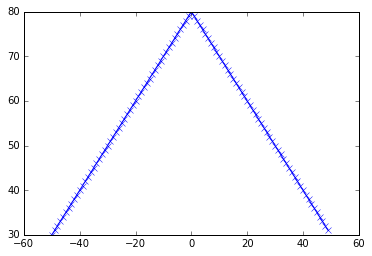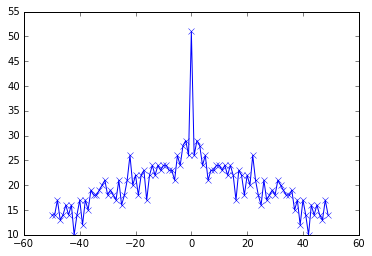It is clear that if you were trying to figure out the sample delay, you are much better off with the faster signal because the it is very obvious when you have the timing right. With the slower signal, you can be a little wrong and still get within a few pc of the correct correlation value.

Code Division Multiplexing¶

Try out CDM for myself. It is important that -1,1 is used instead of 0,1 because multiplying by 0 is always 0.

In :
def gen_code(N):
"""
Generatea a random array containing -1 and 1 of size N
"""
out = np.random.randint(0, 2, N)
out *= 2
out -= 1
return out

def code_modulate(signal, stretch=5, carrier=None):
signal = np.repeat(signal, stretch)
if carrier is None:
carrier = gen_code(len(signal))

return np.multiply(signal, carrier), carrier

sig_1 = gen_code(100)
sig_2 = gen_code(100)

out_1, c_1 = code_modulate(sig_1)

# generate an orthogonal array by flipping every other element
c_2 = np.array(c_1)
c_2[::2] = -1*c_1[::2]
out_2, _ = code_modulate(sig_2, carrier=c_2)

out_comb = out_1 + out_2

def plot_sig(s, title=''):
plt.figure()
plt.stem(s)
plt.ylim([min(s)-0.5, max(s)+0.5])
plt.title(title)

plot_sig(sig_1, 'sig_1')
plot_sig(sig_2, 'sig_2')
plot_sig(out_comb, 'combined output')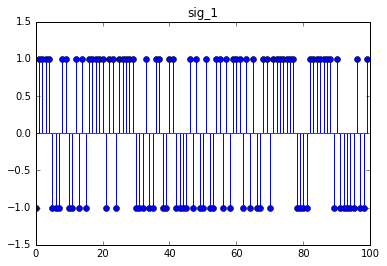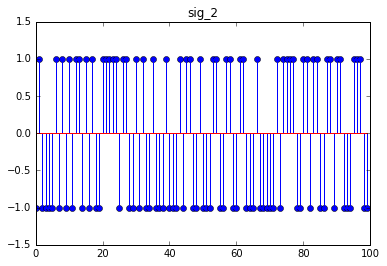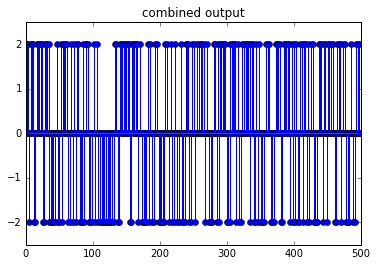In :
def code_demodulate(signal, carrier, stretch=5):
y = np.multiply(signal, carrier)
ysubs = np.split(y, len(y)/5)
ysubs = map(sum, ysubs)
ysubs = map(lambda x:max(-1, x), ysubs)
ysubs = map(lambda x:min(1, x), ysubs)
return ysubs

x = code_demodulate(out_comb, c_1)
plot_sig(x, 'sig_1 recovered')
plot_sig(sig_1, 'sig_1 expected')

x = code_demodulate(out_comb, c_2)
plot_sig(x, 'sig_2 recovered')
plot_sig(sig_2, 'sig_2 expected')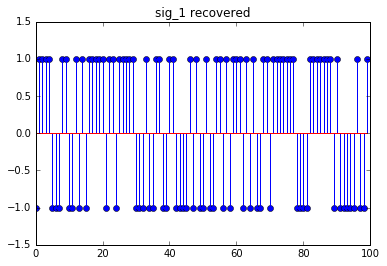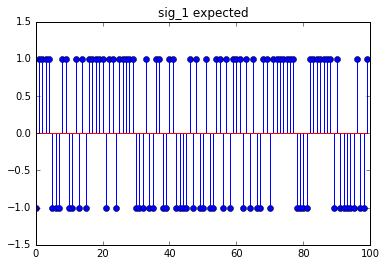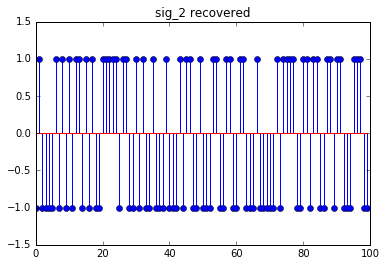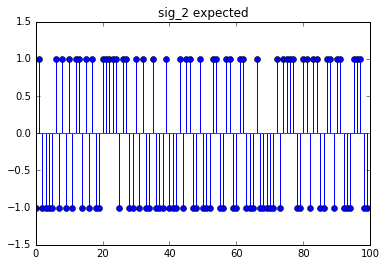Perfect.

In [ ]: